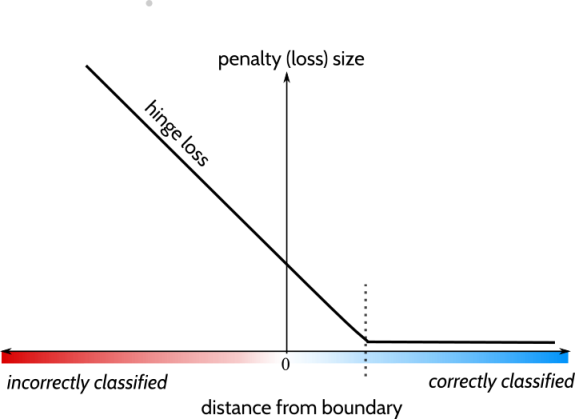DNN 如何调优？

## 欠拟合

• 增加模型深度：ResNet20 -> ResNet50

• 微调结构：更小的 feature map & pooling size

• 更换复杂网络：AlexNet -> ResNet -> …

• DeepCTR 模型使用高阶特征交叉（DCN、xDeepFM）

• 梯度弥散：BatchNorm

## 过拟合

• 数据预处理：不平衡类别；异常值剔除；PCA / ZCA Whitening 或者 BatchNorm；加入噪音增强模型鲁棒性

• 数据扩增：加入噪音；图片旋转、平移、剪裁、颜色、调色；SMOTE；合成样本；使用外部数据

• 迁移学习：在 ImageNet VGG 基础上训练，根据数据分布决定是否冻结权重

• 正则化：L1、L2

• Dropout

• 早停

• 多目标学习：ESMM

• 半监督学习：无标注样本假设（平滑假设、聚类假设、流形假设）

• 迁移学习

• 多模态学习

• 对抗训练：GAN，合成对抗样本

## 精度提升

• 初始化：全0、随机、he_normal() $mean=0,var=sqrt(2/fan\_in)$glorot_normal() $mean=0,var=sqrt(2 / (fan\_in + fan\_out))$
• 激活函数
• 损失函数
• 组合模型：Bagging 或 Boosting；CV；同一模型多次初始化训练
• 样本不平衡：负样本降采样；设置类权重；2-stage 分类任务；单独在少数类别样本上微调
• 特征工程：Embedding

## 附录

### 范数

L1最优化问题的解是稀疏性的, 其倾向于选择很少的一些非常大的值和很多的insignificant的小值. 而L2最优化则更多的非常少的特别大的值, 却又很多相对小的值, 但其仍然对最优化解有显著的贡献. 但从最优化问题解的平滑性来看, L1范数的最优解相对于L2范数要少, 但其往往是最优解, 而L2的解很多, 但更多的倾向于某种局部最优解.

L0范数本身是特征选择的最直接最理想的方案, 但如前所述, 其不可分, 且很难优化, 因此实际应用中我们使用L1来得到L0的最优凸近似. L2相对于L1具有更为平滑的特性, 在模型预测中, 往往比L1具有更好的预测特性. 当遇到两个对预测有帮助的特征时, L1倾向于选择一个更大的特征. 而L2更倾向把两者结合起来.#### L0-范数

$d=\sum_{i=1}^{n}\bigg(x_i^{0}\bigg)^{\frac {1}{0}}$

#### L1-范数

$d=\sum_{i=1}^{n}\bigg|x_i-y_i\bigg|$

#### L2-范数

$d=\sum_{i=1}^{n}\bigg(|x_i-y_i|^{2}\bigg)^{\frac {1}{2}}$

#### Lp-范数

$d=\sum_{i=1}^{n}\bigg(|x_i-y_i|^{p}\bigg)^{\frac {1}{p}}$

#### 无限范数距离

d = \lim_{p \to \infty}\sum_{i=1}^{n}\bigg(|x_i-y_i|^{p}\bigg)^{\frac {1}{p}} = \max(|x_1-y_1|,…,|x_n-y_n|)

### 损失函数

#### 0 - 1 损失函数

gold standard

$L_{01}(m)=\begin{cases} 0 &\text{if } m \ge 0 \\ 1 &\text{else} \end{cases}$

#### 对数损失函数

Log Loss, cross entropy error

$L(Y,P(Y|X))=-\log P(Y|X)$

$P(Y=0|X)=\frac {1}{1+e^{wx+b}}, P(Y=1|X)=1-P(Y=0|X)$

#### 平方损失函数

Square Loss

$L(Y,P(Y|X))=\sum_{i=1}^{n}(Y-f(X))^2$

#### 指数损失函数

Exponential Loss

$L(Y,f(X))=e ^ {-Y f(X)}$

$f_m(x)=f_{m-1}(x)+\alpha_mG_m(x)$

${\arg\ \min}_{\alpha, G}=\sum_{i=1}^{N}e^{[-y_i(f_{m-1}(x_i)+\alpha G(x_i))]}$

#### 合页损失

Hinge Loss, 如 SVM

$L(Y,f(X))=max(0,1-Y * f(X)), Y=\{0,1\}$#### Huber 损失

$L_{\delta}(a) = \begin{cases} \frac{1}{2} a^2 &\text{for } |a| \le \delta,\\ \delta \big( |a| - \frac{1}{2} \delta \big) &\text{otherwise.} \end{cases}$

$L_{\delta}\big(y, f(x)\big) = \begin{cases} \frac{1}{2} \big(y-f(x)\big)^2 &\text{for } \big|y-f(x)\big| \le \delta,\\ \delta \big|y-f(x) \big| - \frac{1}{2} \delta^2 &\text{otherwise.} \end{cases}$### 正则化

Regulization or Penalization：

${\min}_{w \in \Theta}\frac{1}{N}\sum_{i=l}^{N}L(y_i,f(x_i,w))+\lambda J(w)$

$J_0(w) = \big|\{i:w_i \ne 0\}\big|$

$J_1(w) = \sum_i |w_i|$

$J_2(w) = \frac{1}{2} ||w||^2$

$L_1$ 正则项有特征选择功能，能够得到更加稀疏、更多 0 的解。可以用简单截断法，梯度截断法（TG, Truncated Gradient），ADMM 方法求解。

$w\in \mathbb R^2$ 为例，椭圆形是 loss 的损失等高线，灰色区域是约束区域，等高线与约束区域相交的地方，就是最优解。可以看出，L1 较 L2 有更大的概率在角处相交，得到稀疏解。### Batch Norm

Internal Covariate Shift 定义：在深层网络训练的过程中，由于网络中参数变化而引起内部结点数据分布发生变化的这一过程被称作Internal Covariate Shift。

BN 改进了 Whitening 计算耗时的问题，退而求其次，对每个特征进行标准化，使其具有均值为0，方差为1的分布。### 编辑距离

Levenshtein距离：可以删除、加入、取代字符串中的任何一个字元

LCS（最长公共子序列）距离：只允许删除、加入字元

Jaro 距离：只允许字符转置

### Jaccard 距离

$J(A,B) = \frac{A \cap B}{A \cup B}$

### 余弦相似性

$dist(A, B)=\cos\theta =\frac{A \cdot B}{||A||\times||B||}$

0度角的余弦值是1，而其他任何角度的余弦值都不大于1；并且其最小值是-1。从而两个向量之间的角度的余弦值确定两个向量是否大致指向相同的方向。两个向量有相同的指向时，余弦相似度的值为1；两个向量夹角为90°时，余弦相似度的值为0；两个向量指向完全相反的方向时，余弦相似度的值为-1。这结果是与向量的长度无关的，仅仅与向量的指向方向相关。余弦相似度通常用于正空间，因此给出的值为0到1之间。